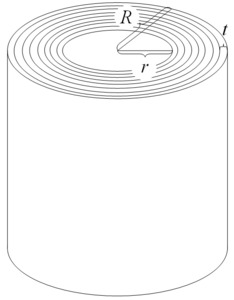# Toilet Roll

Alignments to Content Standards: G-MG.A.1

Picture a roll of toilet paper; assume that the paper in the roll is very tightly rolled. Assuming that the paper in the roll is very thin, find a relationship between the thickness of the paper, the inner and outer radii of the roll, and the length of the paper in the roll. Express your answer as an algebraic formula involving the four listed variables.

## IM Commentary

The purpose of this task is to engage students in geometric modeling, and in particular to deduce algebraic relationships between variables stemming from geometric constraints. The modelling process is a challenging one, and will likely elicit a variety of attempts from the students. Teachers should expect to spend time guiding students away from overly complicated models. Similarly, the task presents one solution, but alternatives abound: For example, students could imagine slicing the roll along a radius, unraveling the cross-section into a sequence of trapezoids whose area can be computed.

## SolutionWe begin by labeling the variables, for which the above diagrams may be useful. Let $t$ denote the thickness of the paper, let $r$ denote the inner radius, let $R$ denote the outer radius and let $L$ denote the length of the paper, all measured in inches. We now consider the area $A$, measured in square inches, of the annular cross-section displayed at the top of the first image, consisting of concentric circles. Namely, we see that this area can be expressed in two ways: First, since this area is the area of the circle of radius $R$ minus the area of the circle of radius $r$, we learn that $A = \pi(R^2-r^2)$.

Second,iIf the paper were unrolled, laid on a (very long) table and viewed from the side, we would see a very long thin rectangle. When the paper is rolled up, this rectangle is distorted, but -- assuming $r$ is large in comparison to $t$ -- the area of the distorted rectangle is nearly identical to that of the flat one. As in the second figure, the formula for the area of a rectangle now gives $A=t\cdot L$.

Comparing the two formulas for $A$, we find that the four variables are related by: $$t\cdot L = \pi(R^2-r^2).$$